# Some tiles associated with the 6th unit cubic Pisot number

## Symmetric Tile

One of the dissection polynomials for the 6th unit cubic Pisot is 2c+c3=1, so there is the possibility of a order 3 symmetric tile, with the smaller element at the centre, and the other two elements symmetrically flanking it. On investigation this tile is found to exist.As this tile has c2 symmetry it is 8- (23-)fold degenerate - each of the three elements can be placed in one of two orientations. For the same reason the grouped element technique can be used to generate 2 demi-symmetric tiles.

There are obviously many additional dissections with more elements, include some symmetric dissections. An order 5 version (2c+2c4+c6) is formed by dissecting the central element. This gives rise to 10 further demi-symmetric tiles.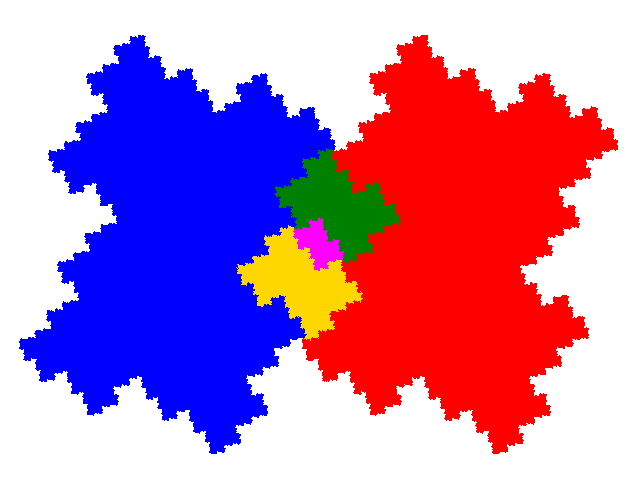Symmetric order 7 versions are formed by dissection of the outer elements of the order 3 version (4c2+c3+2c4) or of the innermost element of the order 5 version (2c+2c3+2c7+c9). The former is the source of an additional 48 demi-symmetric tiles, and the latter of an additional 52 demi-symmetric tiles.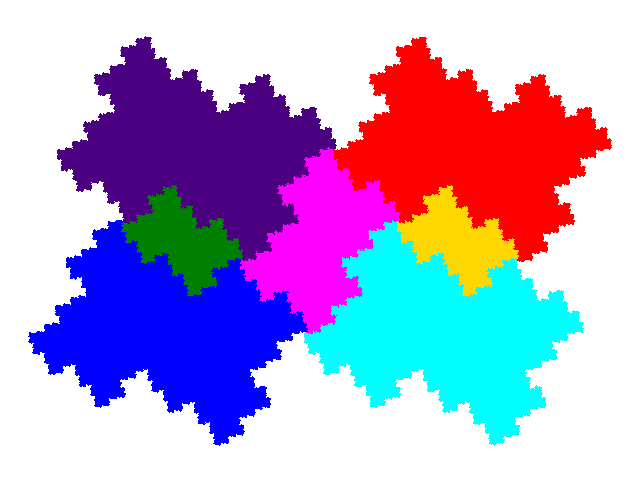Symmetric order 9 versions are formed by dissection of all elements of the order 3 version (4c2+4c4+c6), by the dissection of the two intermediate elements of the order 5 version (2c+4c5+c6+2c7) or by dissection of the innermost element of the order 7 version (2c+2c4+2c7+2c9+c12). These would give rise to hundreds of order 9 demisymmetric tiles.

#### Tiling

There is a great (uncountably infinite) number of tilings of the plane by the symmetric tile, with one or more copies, of various sizes, of the tile forming the unit cell.

If the IFS is ```{ T1:p → ap - 1; T2:p → ap + 1; T3:p → a3p }``` then the obvious candidate values for the tiling vectors are `4` and `4a`. However this leads to a tiling with 6 elements in the unit cell (signature 033344 or 03342). Shuffling copies to find a tiling with a single copy in the unit cell (signature 0) I found that the tiling vectors were ```4(1 + a3 + a6 + a9 + …```) and ```4a(1 + a3 + a6 + a9 + …```). These geometric series sum to `4/(1-a3)` and `4a/(1-a3)`. These numbers can be rewritten as ```3 - a - a2``` and `a(3 - a - a2)`.

There are several ways of notating tilings. For example, as above the signature (the relative sizes of the copies in the cell), in either longhand (e.g. 033) or shorthand (e.g 032) form, can be used, with the offsets given in brackets in the cases where there are multiple tilings for a unit cell. But the signature is not always unique. For example there are two tilings with signature 00111. In this case this can be resolved by dividing the tiling into columns, and ordering the copies in column order, giving 00111 and 01011, repeating for each row. A middle dot can be used to delimit groups of copies within a unit cell, e.g. 0·353 representing a dissection of the smaller element of the 0·2 tiling.

An alternative notation is to use the tiling vectors. But this breaks down when additional tilings are created by dissecting one or more copies in the unit cell, as the tiling vectors are unchanged by such. Even the combination of signature and tiling vectors is not unique, as there are different dissections of the symmetric tile with the same signature. It may be that nothing less than listing the cell transforms (the affine transforms mapping a largest copy in the unit cell to the other copies in the unit cell) and tiling vectors is sufficient, but the vector element of cell transforms can be quite long winded, so this is not exactly convenient. For the time being I will use signatures, and note when they are not unique.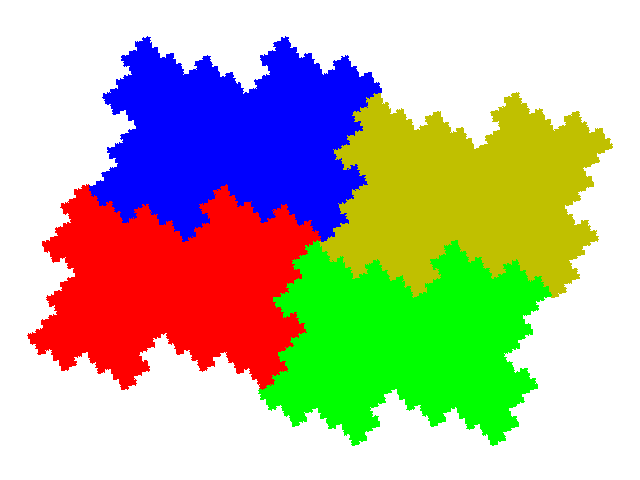There are six ways of creating additional tilings.

• Firstly, the rows and/or columns in the tiling above can be pulled apart, and smaller copies of the tile inserting in the resulting gaps. It is not necessary to pull apart every row or every column - instead every second or every third or so on column, or the second and third columns of each group of three, or so on. To describe the tiling vectors for these it is convenient to define `an = 4an`, and `tn = 4an/(1-a3)` .
• Secondly, in one of the above tilings a copy of the tile can be dissected into smaller copies, or in some cases combined into larger copies.
• Thirdly, in some cases successive rows can be slid rightwards (or leftwards) (`±(t0+t2`)), or successive columns slid upwards (or downwards) (`±(t1+t3`)). In some cases this results in a tiling with the same unit cell, but different tiling vectors, but more often then not moving rows (columns) in the right direction opens up a gap which has to be filled in by additional copies. Furthermore in some tilings (e.g. 00131 and 0131131) successive rows can be slid rightwards (or leftwards) (`±t0`), or successive columns can be slid upwards (or downwards) (`±t1`), giving tilings with the same unit cell, but different tiling vectors. In these cases the unit cell can be redrawn to give herringbone tilings.
• Fourthly, where there are tilings differing only in the offsets between successive rows and columns, such as 03(0) and 03(a0/2), tilings with larger numbers of copies in the unit cell can be created by repeating patterns of the relative positions of rows. For example there is the 4 copies per unit cell tiling 0232(0,a0/2) and the six copies per unit cell tilings 0333(0,0,a0/2) and 0333(0,a0/2,a0/2) represent tilings with 6 copies in the unit cell.
• Fifthly, a tiling can be divided up into unit cells in different ways - a copy can be moved from one side of the central copy to the other.
• Sixthly, there are groups of copies (e.g. 456) which can be arranged in several ways to fill the same space.

Two row tilings exist when the rows are pulled apart by `t2` (02), `-t3` (022), `-t4` (0344), `t6` (05464) and `t5+t6` (0445364); possibly there are more with large numbers of small copies. Three and upwards row tilings exist for sums of these values (and `t1`).

• The three row tiling 0·2·2 is the same as the two row tiling 0·22, except that it is divided into unit cells differently, and might be considered essentially the same tiling.
• The tiling for `t5+t6-t4` (0·342·445364) can be dissected differently to give 0·234455666, which is a sort of 2nd class two row tiling.
• The tiling for `t6-t3` (0·22·5464) is the tiling for `t5+t6`+t5+t6 (0·445364·445364) with many of the copies combined to make two larger copies.
• Similar complications occur for four and greater row tilings.

Two column tilings exist when the columns are pulled apart by `-t2` (01), `-t3` (03), `t4` (033), `t5` (0455), `-t7` (06474) and `-(t6+t7)` (0546374); possibly there are more with large numbers of small copies. Three and upwards row tilings exist for sums of these values (and `t0`).

It can be seen that there are parallels between the two row and two column tilings, which are completed by adding 00 as a two row tiling. (00 is the same tiling as 0, except that pairs of unit cells are arbitarily combined.) For each two row tiling there is a corresponding two column tiling, with the tilings having the same number of copies in the unit cell, and the copies (except for the first) having the same relative sizes and positions, with all the numbers increased by one, and the signs on the vectors reversed. Consequently analogous complications occur for three and greater numbers of columns.

In both cases there is one case where the unit cell belongs to two different tilings, the second being created from the first by sliding successive columns (rows) upwards (rightwards). However the cases are not the corresponding tilings, being the two row tiling 0344 and the two column tiling 03.

 signature: 01 tiling vectors: `t0 - t2` and `t1` cell transforms: `C1:p→p` ```C2:p→ap + (t0 - t2) / 2```signature: 02 tiling vectors: `t0` and ```t1 + t2``` cell transforms: `C1:p→p` ```C2:p→ap + (t1 + t2) / 2```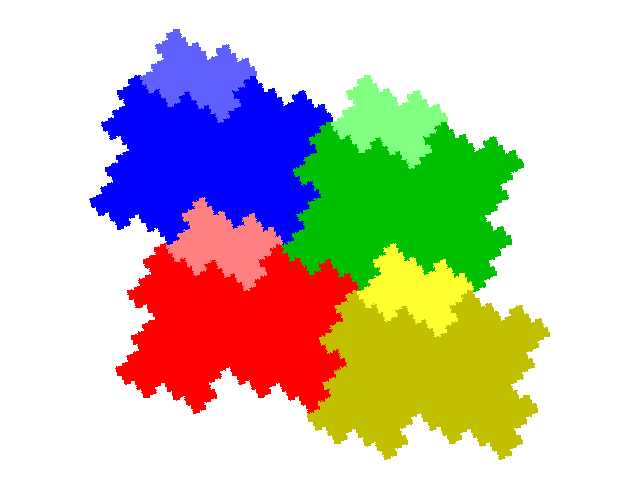signature: 03(0) tiling vectors: ```t0 - t3 (= a0 = 4)``` and `t1` cell transforms: `C1:p→p` ```C2:p→a3p + (t0 - t3) / 2```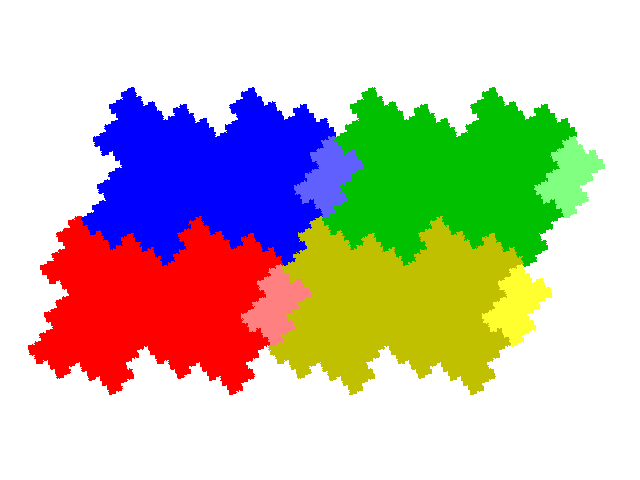signature: 03(a0/2) tiling vectors: ```t0 - t3 (= a0 = 4)``` and ```t1 + t0 + t2``` cell transforms: `C1:p→p` ```C2:p→a3p + (t0 - t3) / 2```signature: 022 tiling vectors: `t0` and ```t1 - t3``` cell transforms: `C1:p→p` ```C2:p→ap + (t1 - t3) / 2 + (t2 + t3) / 2``` ```C3:p→ap + (t1 - t3) / 2 - (t2 + t3) / 2```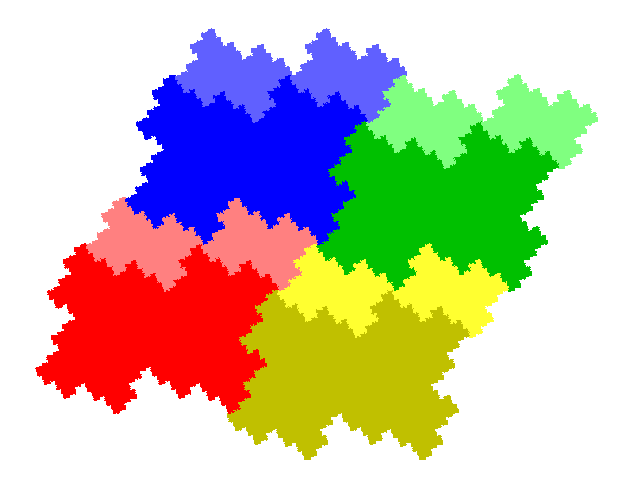signature: 033 tiling vectors: `t0 + t4` and `t1` cell transforms: `C1:p→p` ```C2:p→a3p + (t0 + t4) / 2 + (t3 + t4) / 2``` ```C3:p→a3p + (t0 + t4) / 2 - (t3 + t4) / 2```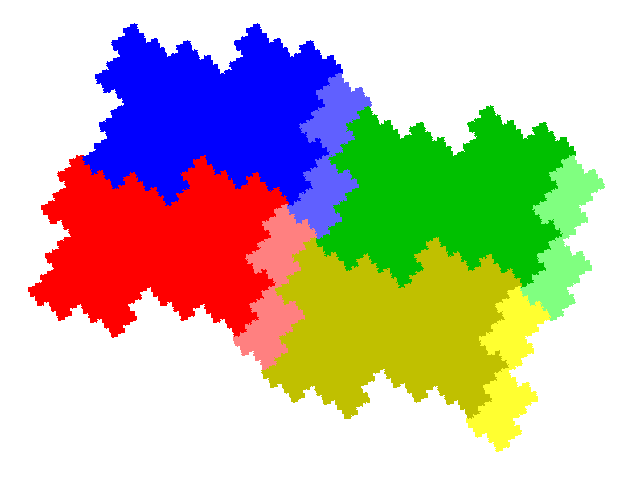signature: 0344(0) tiling vectors: `t0` and ```t1 - t4``` cell transforms: `C1:p→p` ```C2:p→a3p + (t1 - t4) / 2``` ```C3:p→a4p + (t1 - t4) / 2 + (t0 + t2) / 2``` ```C4:p→a4p + (t1 - t4) / 2 - (t0 + t2) / 2```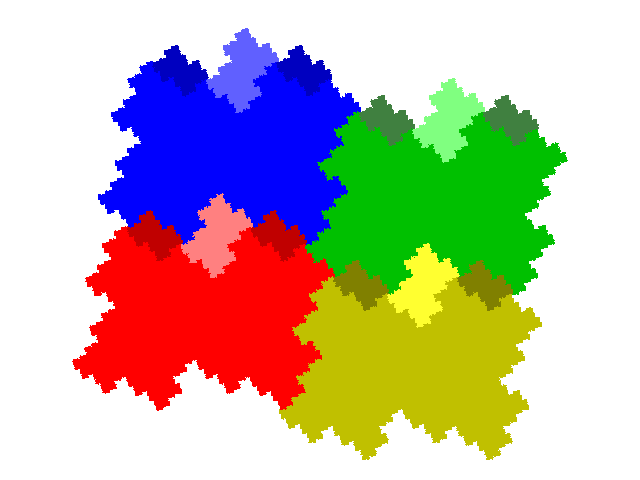signature: 0344(a0/2) tiling vectors: ```t0 + (t1 - t4) / 2``` and ```t1 - t4``` cell transforms: `C1:p→p` ```C2:p→a3p + (t1 - t4) / 2``` ```C3:p→a4p + (t1 - t4) / 2 + (t0 + t2) / 2``` ```C4:p→a4p + (t1 - t4) / 2 - (t0 + t2) / 2```signature: 0455 tiling vectors: `t0 + t5` and `t1` cell transforms: `C1:p→p` ```C2:p→a4p + (t0 + t5) / 2``` ```C3:p→a5p + (t0 + t5) / 2 - (t1 + t3) / 2``` ```C4:p→a5p + (t0 + t5) / 2 + (t1 + t3) / 2```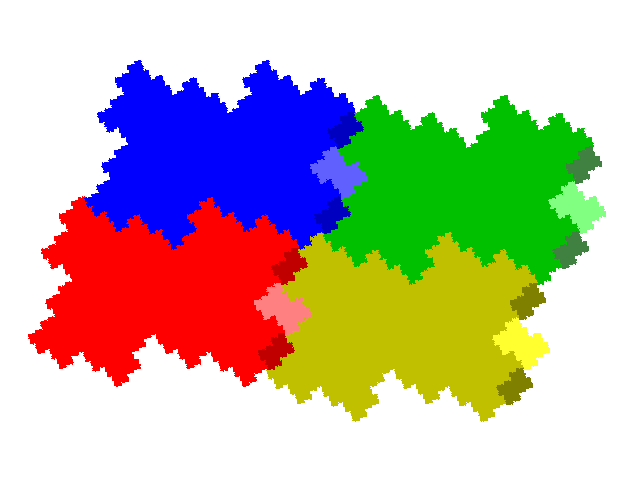signature: 05464 tiling vectors: `t0` and ```t1 + t6``` cell transforms: `C1:p→p` ```C2:p→a5p + (t1 + t6) / 2 + (t0 + t2) / 2``` ```C3:p→a5p + (t1 + t6) / 2 - (t0 + t2) / 2``` ```C4:p→a5p + (t1 + t6) / 2 + (t0 + t2) / 2 + (t2 + t4)``` ```C5:p→a5p + (t1 + t6) / 2 - (t0 + t2) / 2 - (t2 + t4)``` ```C6:p→a6p + (t1 + t6) / 2 + (t0 + t2) / 2 + (t2 + t4) / 2``` ```C7:p→a6p + (t1 + t6) / 2 + (t0 + t2) / 2 - (t2 + t4) / 2``` ```C8:p→a6p + (t1 + t6) / 2 - (t0 + t2) / 2 + (t2 + t4) / 2``` ```C9:p→a6p + (t1 + t6) / 2 - (t0 + t2) / 2 - (t2 + t4) / 2```signature: 06474 tiling vectors: `t0 - t7` and `t1` cell transforms: `C1:p→p` ```C2:p→a6p + (t0 - t7) / 2 + (t4 + t6) / 2``` ```C3:p→a6p + (t0 - t7) / 2 - (t4 + t6) / 2``` ```C4:p→a6p + (t0 - t7) / 2 + (t4 + t3) / 2``` ```C5:p→a6p + (t0 - t7) / 2 - (t4 + t3) / 2``` ```C6:p→a7p + (t0 - t7) / 2 + (t4 - t5) / 2``` ```C7:p→a7p + (t0 - t7) / 2 - (t4 - t5) / 2``` ```C8:p→a7p + (t0 - t7) / 2 + (t4 - t5) / 2 + (t3 + t5)``` ```C9:p→a7p + (t0 - t7) / 2 - (t4 - t5) / 2 - (t3 + t5)```signature: 0445364 tiling vectors: `t0` and ```t1 + t5 + t6``` cell transforms: `C1:p→p` ```C2:p→a4p + (t0 - t5) / 2 + t1 + t3``` ```C3:p→a4p + (t0 - t5) / 2 + t1 + t3 - t0 - t2``` ```C4:p→a4p + (t0 - t5) / 2 + t1 + t3 + t2 + t4``` ```C5:p→a4p + (t0 - t5) / 2 + t1 + t3 - t0 - t2 + t2 + t4``` ```C6:p→a5p + (t1 + t6) / 2 + t5 - (t0 + t2) / 2``` ```C7:p→a5p + (t1 + t6) / 2 + t5 + (t0 + t2) / 2``` ```C8:p→a5p + (t1 + t6) / 2 + t5 + (t0 + t2) / 2 + t2 + t4``` ```C9:p→a6p + (t1 + t6) / 2 + t5 + (t0 + t2) / 2 + (t2 + t4) / 2``` ```C10:p→a6p + (t1 + t6) / 2 + t5 + (t0 + t2) / 2 - (t2 + t4) / 2``` ```C11:p→a6p + (t1 + t6) / 2 + t5 - (t0 + t2) / 2 + (t2 + t4) / 2``` ```C12:p→a6p + (t1 + t6) / 2 + t5 - (t0 + t2) / 2 - (t2 + t4) / 2```signature: 0546374 tiling vectors: ```t0 - t6 - t7``` and `t1` cell transforms: `C1:p→p` ```C2:p→a5p + (t0 - t6) / 2 + (t4 - t5) / 2``` ```C3:p→a5p + (t0 - t6) / 2 - (t4 - t5) / 2``` ```C4:p→a5p + (t0 - t6) / 2 + (t4 - t5) / 2 + (t3 + t5)``` ```C5:p→a5p + (t0 - t6) / 2 - (t4 - t5) / 2 - (t3 + t5)``` ```C6:p→a6p + (t0 - t6) / 2 - t6 - t7``` ```C7:p→a6p + (t0 - t6) / 2 - t6 - t7 + (t3 + t5)``` ```C8:p→a6p + (t0 - t6) / 2 - t6 - t7 - (t3 + t5) - (t4 + t5 + t7 + t8) / 2``` ```C9:p→a7p + (t0 + t5) / 2 - (t3 + t5)``` ```C10:p→a7p + (t0 + t5) / 2``` ```C11:p→a7p + (t0 + t5) / 2 + (t3 + t5) - t6 - t7``` ```C12:p→a7p + (t0 + t5) / 2 + 2(t3 + t5) - t6 - t7```Given two row (column) tilings, three row (column) tilings are generally easier to generate. One can take a tiling, and add the last rows (columns) of the same or another tiling. The modifications to tiling vectors and to the cell transforms for the last rows (columns) are relatively simple to identify.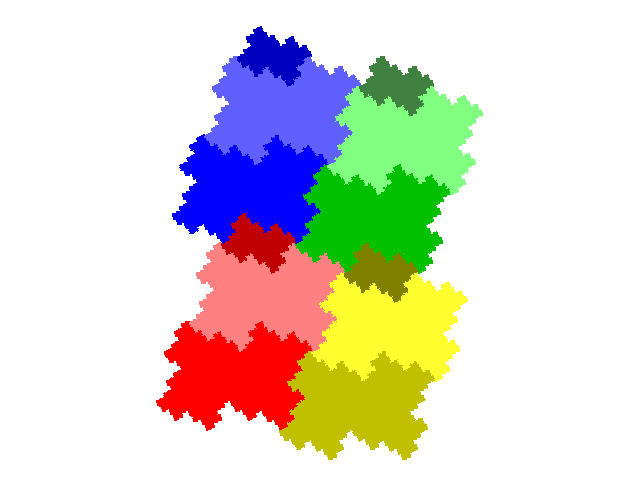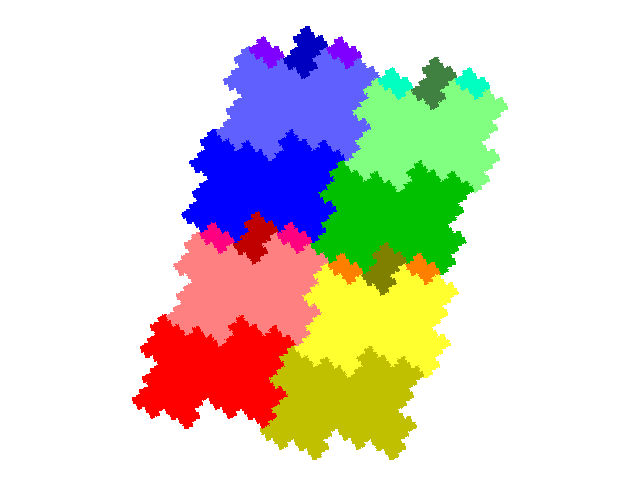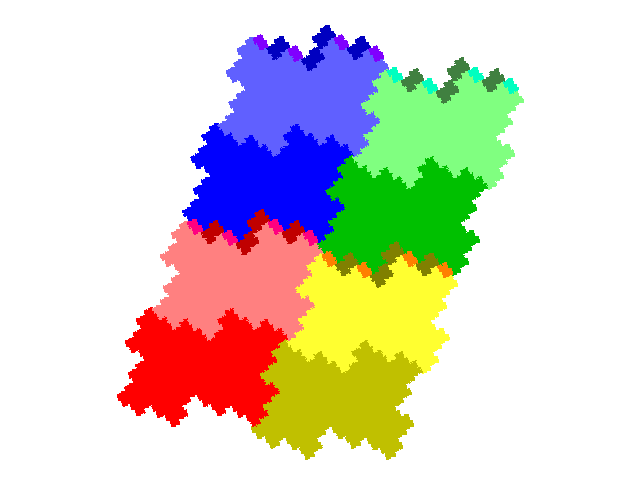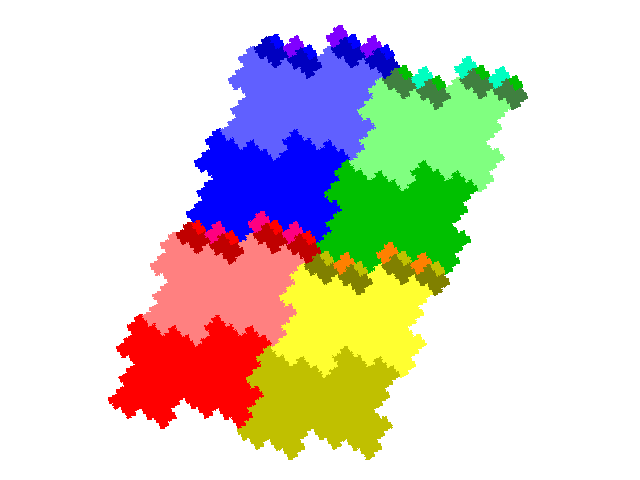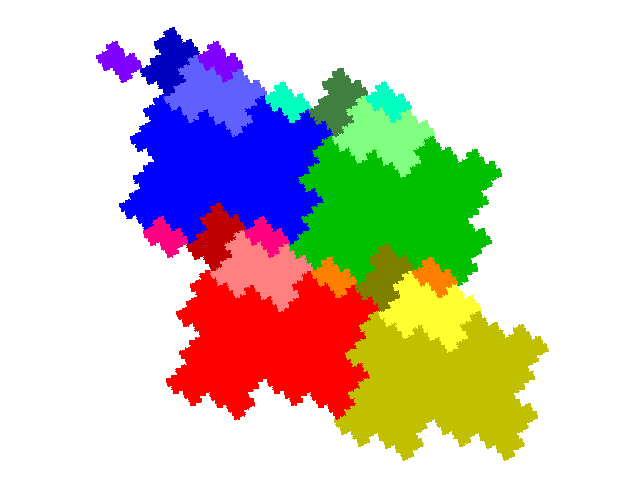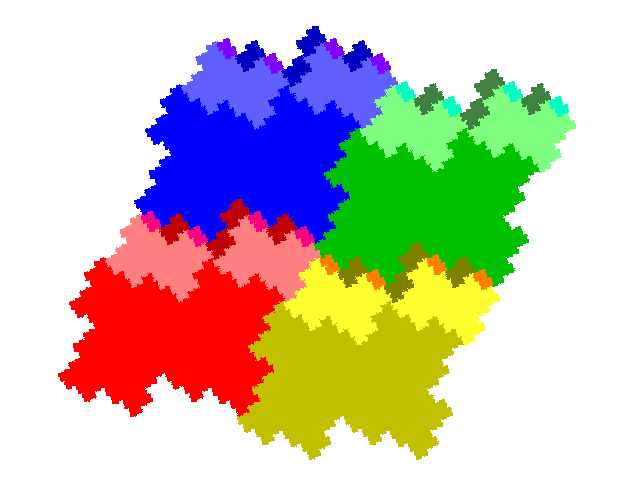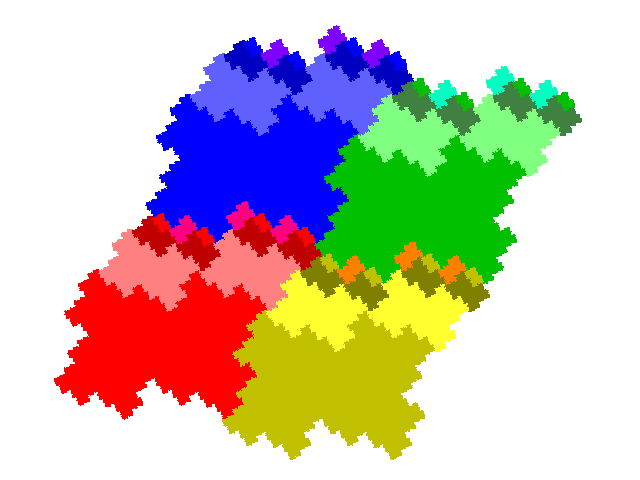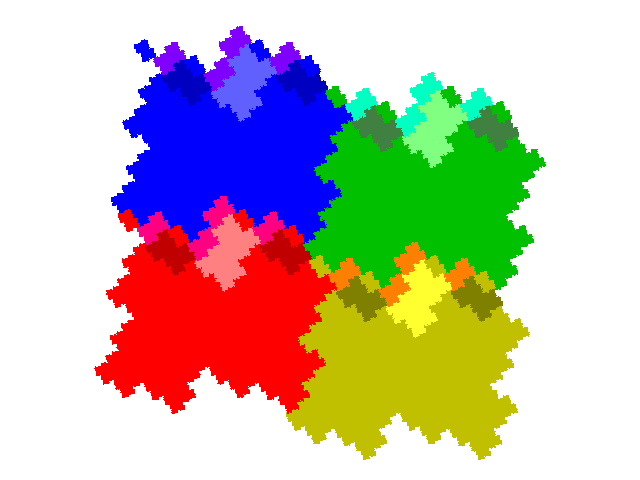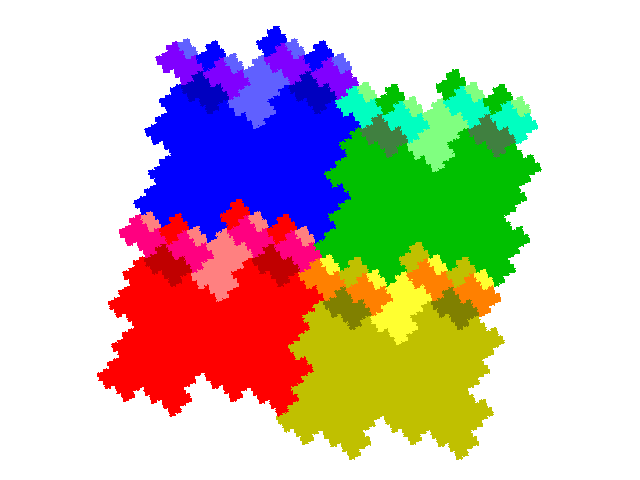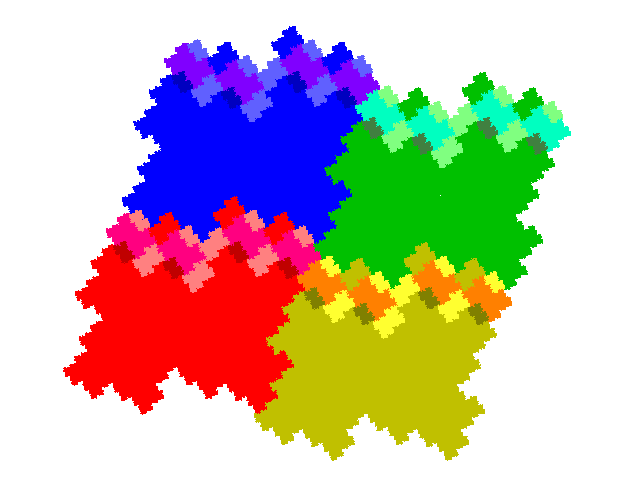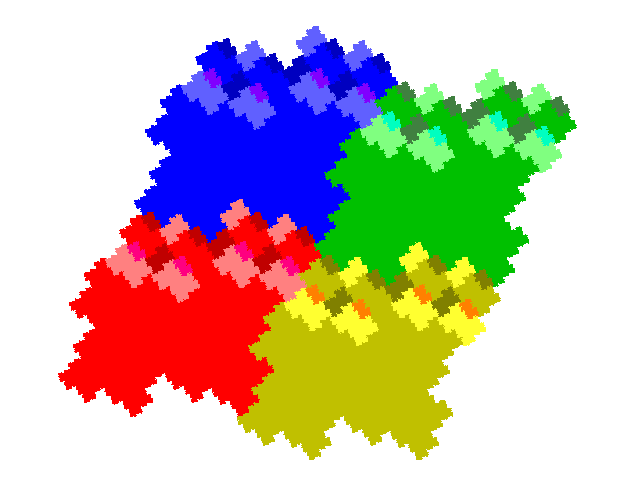The three column tilings are 001, 003, 0033, 00455, 006474, 00546374, 011, 013, 0133, 01455, 016474, 01546374, 0333, 03455, 036474, 03546374, 034, 033455, 0336474, 033546374, 0445455, 04556474, 04566374, 064746474, 06474546374 and 0546374546374.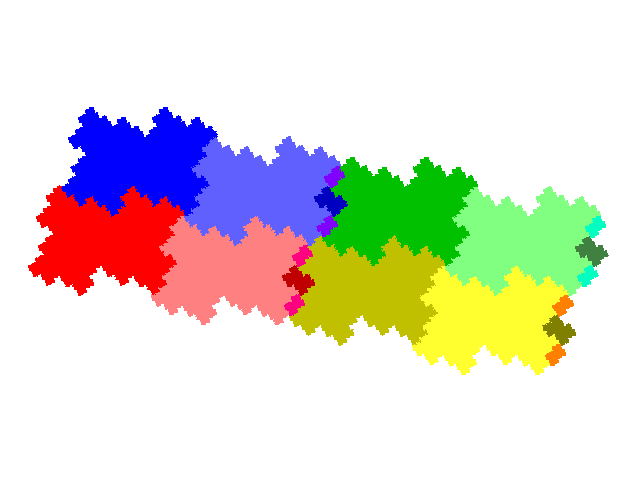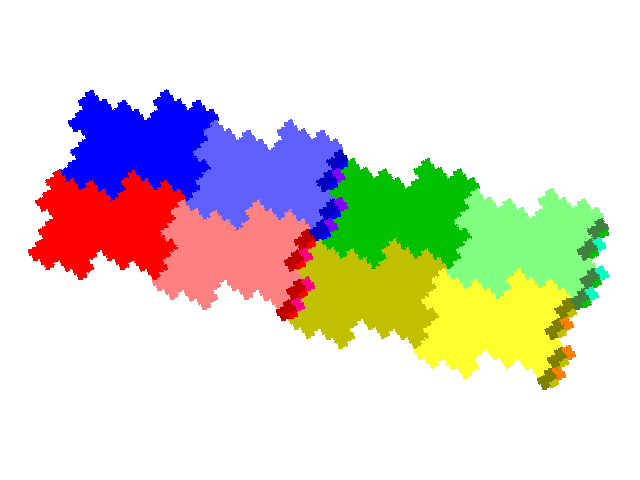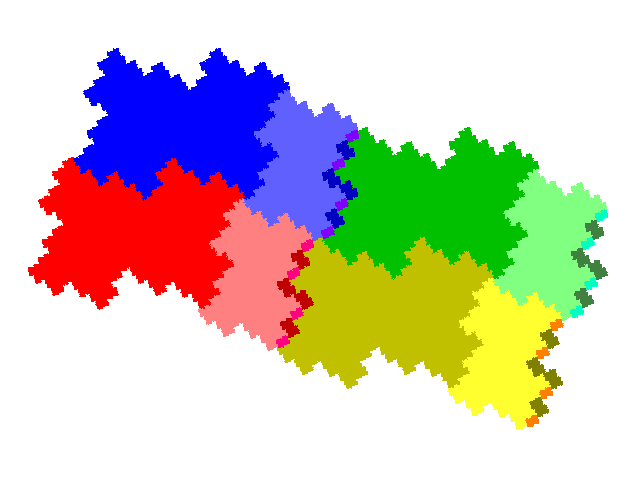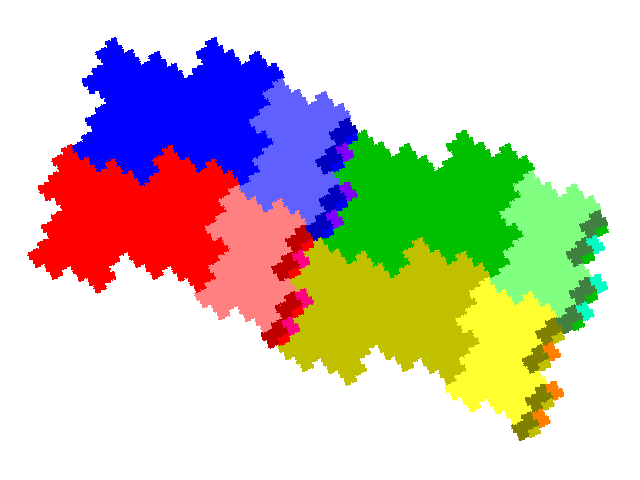,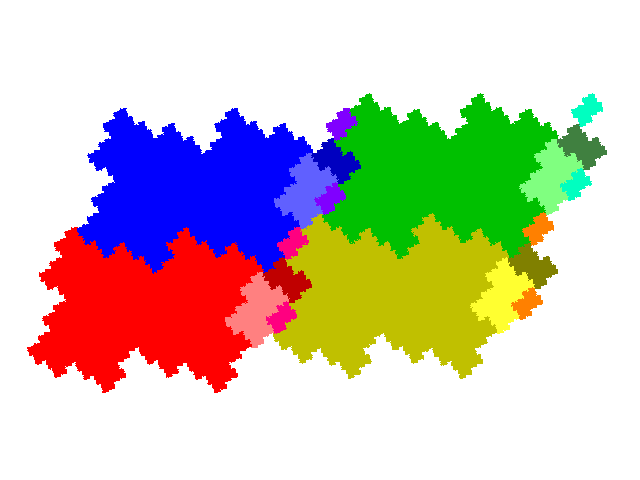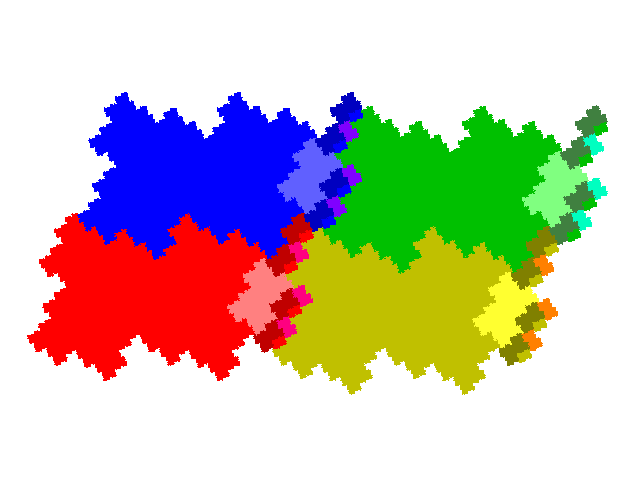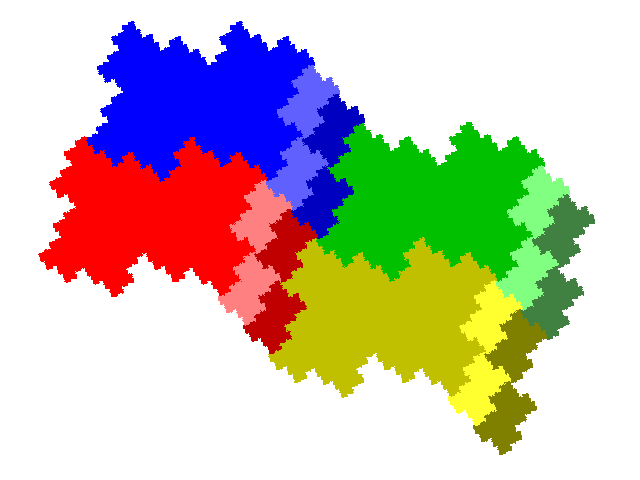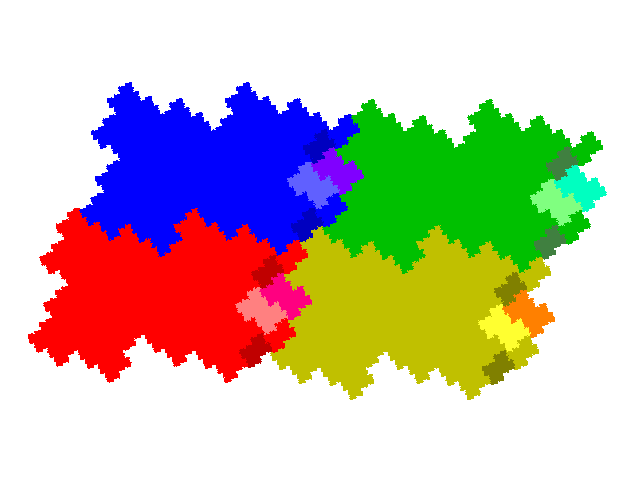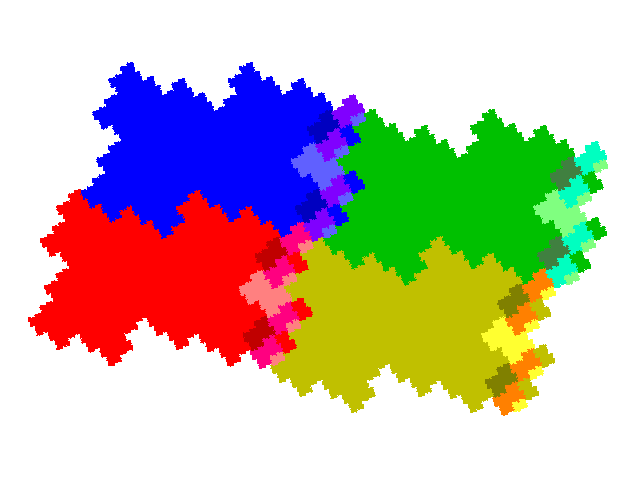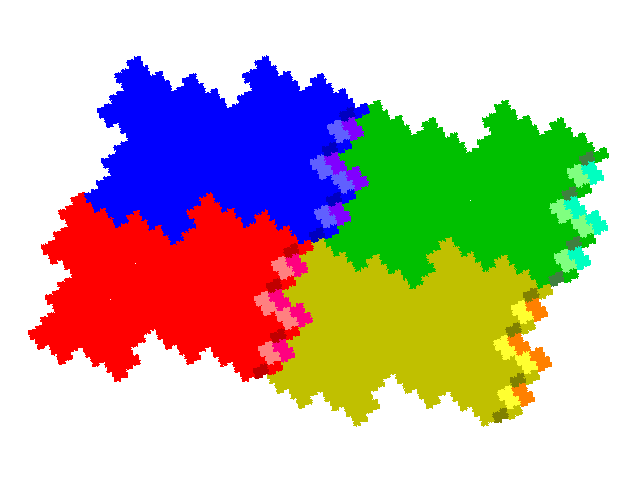In most cases simultaneously pulling both the rows and columns by the vectors identified above generates new tilings, composed of the union of the elements of the two row and two column tilings, with any gaps filled in by additional copies of the tile. In three cases there is an overlap (in which case to generate a tiling one has to dissect an copy and remove one of the resulting copies), and in two pairs of cases, once the gap has been filled in the same tiling results. The signatures of the resulting tiles are shown in the table immediately below.

 01 03 033 0455 06474 0546374 02 012 023·2 02455·44 022 0122·2 0223 02233·3 022455·5 026474·677 022546374·55677 0344 01344·4 03344·3 033344 0344455·4 03446474·66 0344546374·55677 05464 015464·566 035464·44 0335464·6 04555464·5 054646474·6 05464546374·6 0445364 01445364·44566 03445364·5 033445364·46 0455445364·5 04453646474·67 0445364546374·567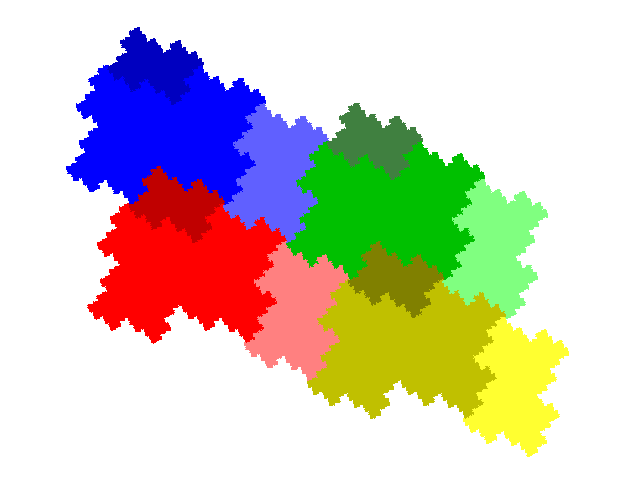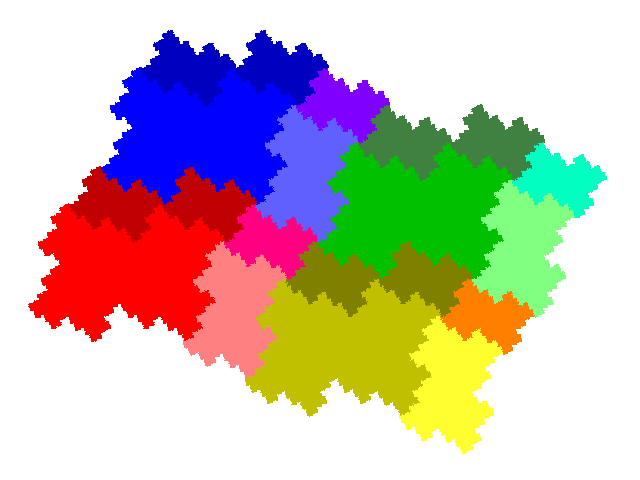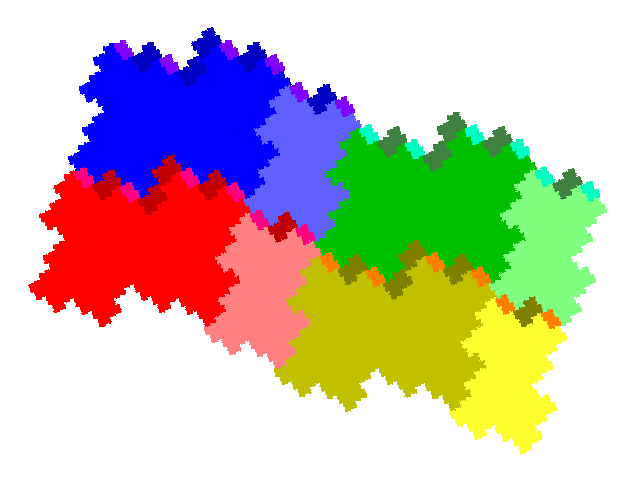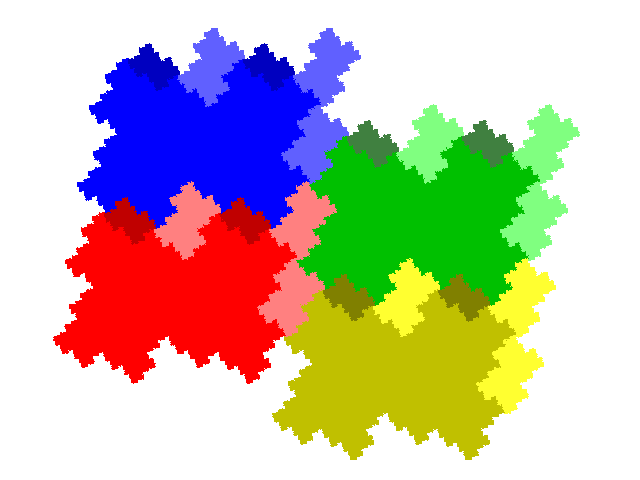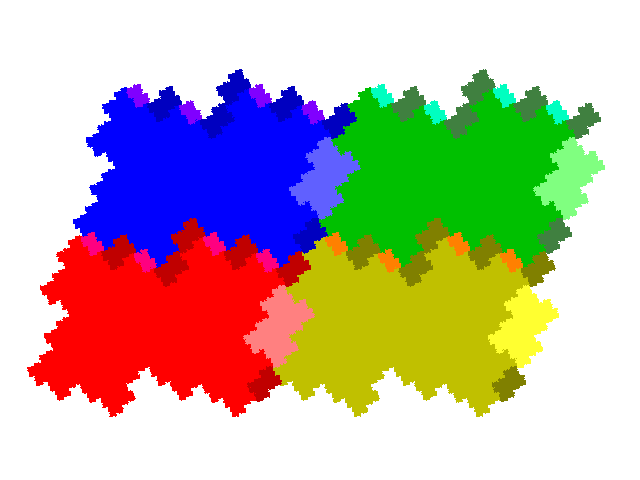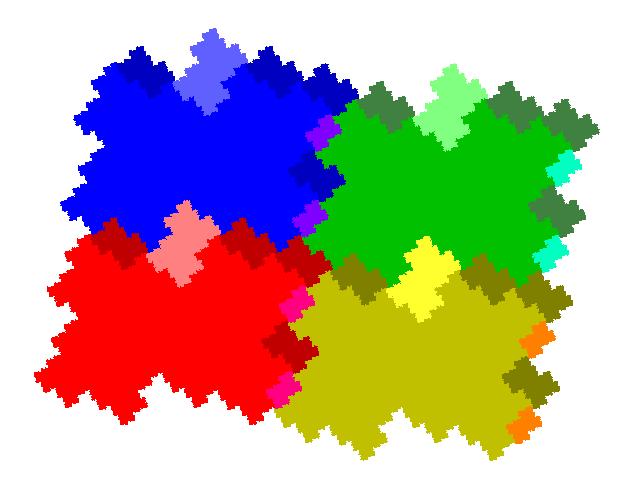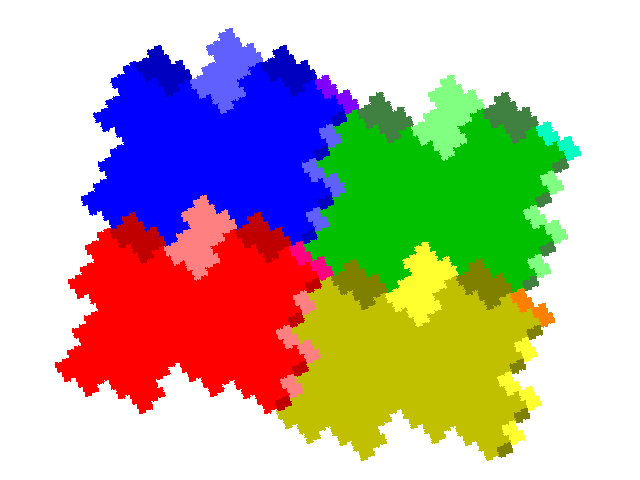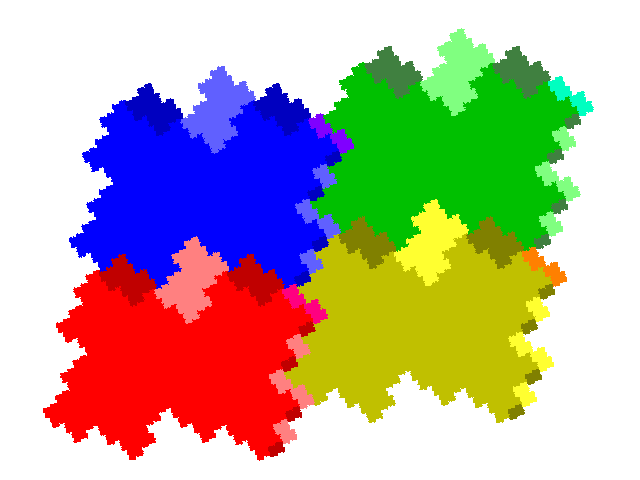Once "2-row × 2-column" tilings have been produced they can be readily generalised to produce "m-row × n-column" tilings.